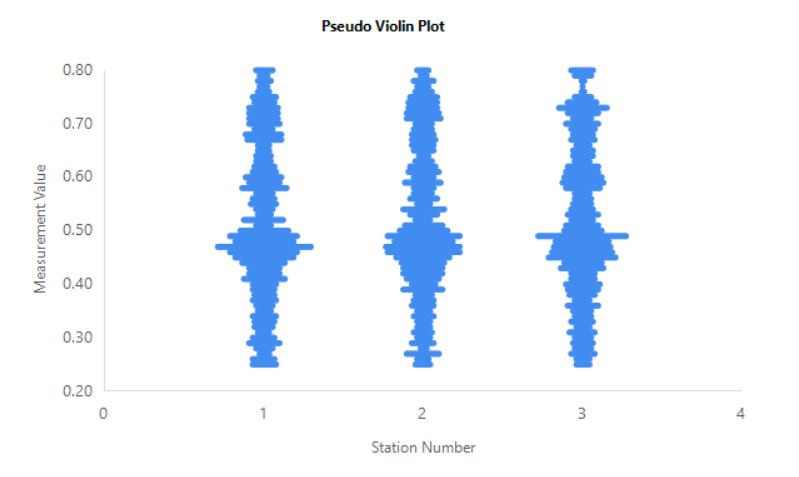# SSRS - Violin Plot

A couple years ago, I received a request to build an SSRS report which would display a violin style plot. In this case, close enough would suffice. It didn’t need to implement a violin plot exactly. The purpose was to visualize a set of pressure measurements across a set of stations.

Violin plots are similar to box plots, except that they also show the probability density of the data at different values, usually smoothed by a kernel density estimator.

## Basic Query

 `````` 1 2 3 4 5 6 7 8 9 10 11 12 13 14 15 16 `````` ``````WITH cte1 AS ( SELECT ID, StationNumber, Measurement, RANK() OVER(PARTITION BY Measurement, StationNumber ORDER BY ID) AS RankOrderMeasurement FROM @data ) SELECT cte1.ID, cte1.StationNumber + ((cte1.RankOrderMeasurement - 1) * (0.3 / t2.MaxRank) * IIF(cte1.RankOrderMeasurement % 2 = 0, -1, 1)) AS X, cte1.Measurement AS Y FROM cte1 CROSS APPLY (SELECT MAX(cte1.RankOrderMeasurement) AS MaxRank FROM cte1) AS t2; ``````

## Design Notes

The y-axis simply plots the actual measurement value.

The x-axis is where the “magic” happens. This assumes stations are integer style numbers, preferably consecutative integers. However, the concept would still work with non-consecutive integers, the graph just might not be as pretty.

The formula in the query to calculate the X value is cte1.StationNumber + ((cte1.RankOrderMeasurement - 1) * (0.3 / t2.MaxRank) * IIF(cte1.RankOrderMeasurement % 2 = 0, -1, 1)) AS X

I want the values for Station Number N to be centered over the number N on the x-axis thus the first piece StationNumber + (STUFF).

The 0.3 factor means the values can be spread from approximately N - 0.3 to N + 0.3 on the x-axis for Station Number N. I want the Y value with the most points to be the widest part of the violin plot. The modulus of 2 is to plot points alternating on the left side and right side of N.

## Sample Output